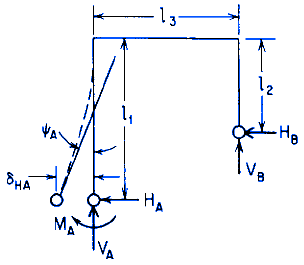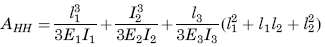Related Resources: calculators

### Concentrated Moment Left Vertical Deflections Equation and Calculator

Beam Deflection and Stress Equation and Calculators

Frame Deflections with Concentrated Moment Applied to Left Vertical Member Equations and Calculator.General Designations

ALL calculators require a Premium Membership

Preview

General reaction and deformation expressions with right and left ends pinned

Horizontal Deflection at A:Reaction locations are pinned therefore, the displacements = 0 = δHA

Angular Rotation at A:Where:Reaction loads and moments VA and VB, and HB can be evaluated from equilibrium equations after calculating HA and MA.Reaction locations are pinned therefore, the Moments = 0 = MA = MB

Where:

Δo = Displacement (in, mm),
W = Load or Force (lbsf, N),
w = Unit Load or force per unit length (lbs/in2, N/mm2)
Mo = Applied couple (moment) ( lbs-in, N-mm),
θo = Externally created angular displacement (radians),
Δo, = Externally created concentrated lateral displacement (in, mm),
T - To = Uniform temperature rise (Deg.),
T1, T2 = Temperature on outside and inside respectively (degrees),
HA, HB = Horizontal end reaction moments at the left and right, respectively, and are positive clockwise (lbs, N),
I1, I2, and I3 = Respective area moments of inertia for bending in the plane of the frame for the three members (in4, mm4),
E1, E2, and E3 = Respective moduli of elasticity (lb/in2, Pa) Related: Modulus of Elasticity, Yield Strength;
γ1, γ2, and γ3 = Respective temperature coefficients of expansions unit strain per. degree ( in/in/°F, mm/mm/°C),
l1, l2, l3 = Member lengths respectively (in, mm),

References:

Roark's Formulas for Stress and Strain